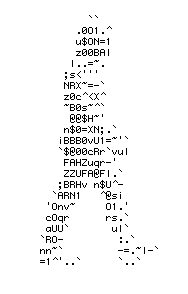Programming  MA 1603    (Andreas Fring)      course syllabusPart II

# Part I

## Lecture notes:

• Complete lecture notes of part I pdf
• Lecture 1 (Basic Excel Terminology and Features):      pdf    handout
• Lecture 2 (Basic Excel Operations)                                 pdf
• Lecture 3 (Built-in functions):                                             pdf    Excel
• Lecture 4 (Built-in function 2, User Defined Functions)  pdf
• Lecture 5 (User Defined Functions)                                 pdf     Excel
• Lecture 6 (If structure)                                                        pdf     Excel
• Lecture 7 (An example tutorial)                                         pdf     Excel
• Lecture 8 (Select case structure)                                      pdf     Excel
• Lecture 9 (Charts)                                                               pdf     Excel
• Lecture 10 (Revisions)                                                        pdf

• Preliminary Exercise:    pdf                     Solutions:   pdf     Excel
• Labsession 1:                pdf                     Solutions:   pdf     Excel
• Labsession 2:                pdf    (Ptable)   Solutions:   pdf     Excel
• Labsession 3:                pdf                     Solutions:   pdf     Excel
• Labsession 4:                pdf                     Solutions:   pdf     Excel
• Labsession 5:                pdf                     Solutions:   pdf     Excel
• Labsession 6:                pdf                     Solutions:   pdf     Excel
• Labsession 7:                pdf                     Solutions:              Excel
• Labsession 8:                pdf                     Solutions:   pdf      Excel

### Tutorial groups:

 Monday    9:00 - 10:50 room A416 ActScience group P1  (E. Lawal) Monday    9:00 - 10:50 room A231 ActScience group P2  (Y. Valani) Thursday   9:00 - 10:50 room A416 ActScience group P3  (G. Bowtell) Friday     15:00 - 16:50 room A416 ActScience Friday     15:00 - 16:50 room A231 ActScience Friday       9:00 - 10:50 room A231 Mathematics group A  (E. Lawal) Friday       9:00 - 10:50 room  A416 Mathematics group B  (S. Mehta) Friday       9:00 - 10:50 room A416 Mathematics group C  (Y. Valani)